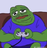首页   注册   登录
V2EX = way to explore
V2EX 是一个关于分享和探索的地方

V2EX  ›  算法

# 关于快排 quicksort 的两种写法

Alpacino · 120 天前 · 528 次点击

``````def partition(arr,low,high):
i = low      # index of smaller element
pivot = arr[high]     # pivot
for j in range(low , high):
if   arr[j] <= pivot:
arr[i],arr[j] = arr[j],arr[i]
i = i+1
arr[i],arr[high] = arr[high],arr[i]
return i

def quickSort(arr,low,high):
if low < high:
pi = partition(arr,low,high)
quickSort(arr, low, pi-1)
quickSort(arr, pi+1, high)
``````

``````def partition(arr,low,high):
i = ( low-1 )         # index of smaller element
pivot = arr[high]     # pivot

for j in range(low , high):
if   arr[j] <= pivot:
i = i+1
arr[i],arr[j] = arr[j],arr[i]

arr[i+1],arr[high] = arr[high],arr[i+1]
return ( i+1 )

def quickSort(arr,low,high):
if low < high:
pi = partition(arr,low,high)
quickSort(arr, low, pi-1)
quickSort(arr, pi+1, high)
``````
2 回复  |  直到 2019-07-16 15:14:24 +08:001 Alpacino   120 天前 这两个写法有什么区别吗？是处理 edge case 上的不同？2 billc   119 天前 茴香豆的茴有 4 种写法。--------------------------------------------正经回答：没差别，你把下面所有的 i，替换成 i-1，和上面的完全一样